Up | CircleFinder2D - 165 | EasyOCR - 78 | PolygonMatch2 - 84 | GCPS -110 | HeightMap3D - 111 | ImageExtrema - 129 | PCA3D - 140 | CameraPose3D - 148 | MonoPose3D - 149 | ImagePicker3D - 145 | ExternalCoordinates3D - 157 | MaMa3D - 152 | CircleSegmentor - 153 | PolygonMatch3 - 160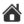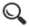CameraPose3D - 148

The CameraPose3D can dynamically or manually locate the pose of a camera.

The tool is normally used in conjuction with

The design target for this tool was to be able to dynamically calculate the pose of up to four cameras in system where a 3D model is calculated based on four camera and a laser grid. The CPC - CreatePointCloud3D - calculates the 3D model.

The image below illustrate how six balls with known 3D position - x,y,z - can be used to calculated the six degree of freedom for the camera.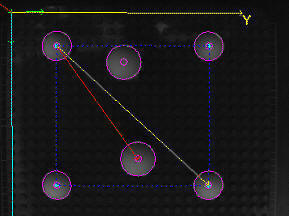The tool is replaced by MonoPose3D.

Setup

3D Reference tools (one per camera image) - 3D Reference system selection

• An ExternalReference3D tool instance per camera(or None if not in use)

Points - up to 12 points

These are 2D tool results connected to its corresponding 3D position.

• Active - toggle calculation of this point

Camera point

• Tool - tool that reports a point
• Result - which point result to use

Object point

• x,y,z coordinates given in the 3D coordinate system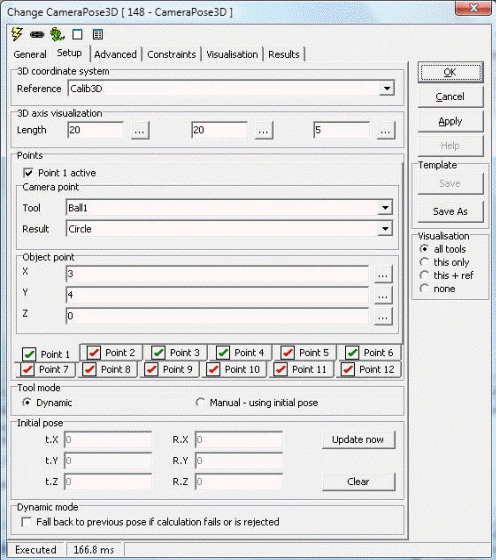Constraints

• Max deviation for accepted point - acceptance criterion for each point (see Deviation above).
• Ignore camera points with deviation ratio over [dB] - ignore cameras where 3D deviation ratio to the best camera is over the threshold - measured in dB
• Ignore camera points with deviation over - used to remove "bad" points before estimating position in space
• Minimum number of active cameras - accept point only if at least this many cameras have a valid result

Constraints for mean point

This is active only if All points the same is checked on the Setup page. All found points are averaged and results taken from there.

• Max deviation (spread) for accepted point - acceptance criterion for the common (mean) point. The spread is calculated in 2D as the maximum distance from the incoming tool points to the mean point.
• Minimum number of accepted points - accept mean point only if at least this many points have a valid result.

Include in on-screen-description - requires description to be active under Visualisation

• Point number - (not available if All points the same is checked)
• 3D Coordinates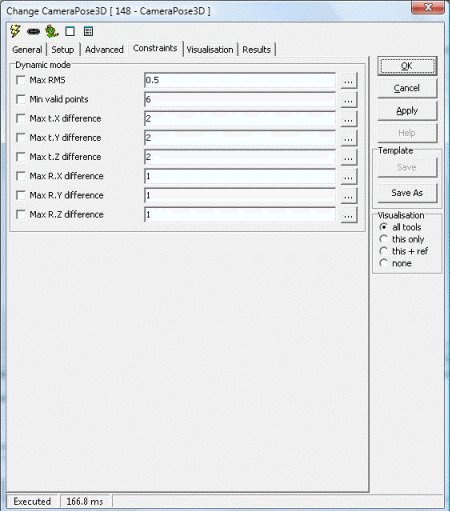Visualisation

 AcceptedPoint Point that passes constraints FailDescription Description of a failed point FailedPoint Found point that fails constraints IncomingPoint Input points from other tools OKDescription Description of an accepted point

Results

 Point[1-8].x X position for found 3D point Point[1-8].y Y position for found 3D point Point[1-8].z Z position for found 3D point Deviation[1-8] Point deviation Cameras used[1-8] Number of used cameras for point Cameras in use[1-8] Python tuple of the cameras used to generate the result Accepted[1-8] Constraints result (0=fail, 1=accepted) Mean point (.x,.y,.z) Mean of found points (only for all points are the same) Spread Max distance from single point to the mean Active points Count of active input points Accepted points Count of points accepted and reported

Example 1: Measure Distance Between Points

```import math

def Distance3D (x1,y1,z1,x2,y2,z2):
return math.sqrt((x1-x2)*(x1-x2) + (y1-y2)*(y1-y2) + (z1-z2)*(z1-z2))

def GetPoint3D(name):
x = GetFloatValue(name+'_x')
y = GetFloatValue(name+'_y')
z = GetFloatValue(name+'_z')
return x,y,z

def SetPoint3D(name,x,y,z):
SetFloatValue(name+'_x',x)
SetFloatValue(name+'_y',y)
SetFloatValue(name+'_z',z)

x1,y1,z1 = GetPoint3D('Points3D1.Point1')
x2,y2,z2 = GetPoint3D('Points3D1.Point2')
x3,y3,z3 = GetPoint3D('Points3D1.Point3')

width = Distance3D(x1,y1,z1,x2,y2,z2)
height = Distance3D(x1,y1,z1,x3,y3,z3)

print 'w,h -',width,height```

 Scorpion Vision Version XII : Build 646 - Date: 20170225 Scorpion Vision Software® is a registered trademark of Tordivel AS. Copyright © 2000 - 2017 Tordivel AS.